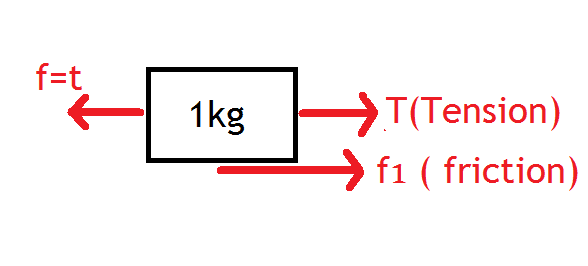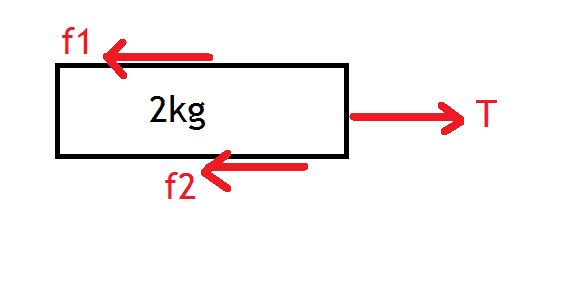# Plotting the graph of Friction Force vs. time (Laws of motion)

• SpectraPhy09

#### SpectraPhy09

Homework Statement
Coefficient of friction between all the surfaces in 0.5. Which diagram would describe the friction between the ground and the 2Kg Block when f = t N is applied on the 2kg Block? It is given that the string is not taut initially.(Plz check the the diagram in the image attached). take g= 10m/s^2
Relevant Equations
F = ma
F(friction) = μmg
F.B.D Of first block
(I have shown only the horizontal Forces)f1(max) = μ (1kg)(g) = 0.5 * 10 = 5N
F.B.D Of the second Blockf2(max) = μ (3kg)(g) = 15N

Now the string will become taut and the tension will start acting when f = t = 5N
But for 0<f<5N there will be no motion between the 1 kg and the 2 kg block so the f2=0
but when f > 5N the string will become taut and the friction the Tension will start will acting now suppose the acceleration of the 1 kg block in left direction is a1 then,
(1)a1 = f - T - f1
and suppose the acceleration od the 2kg block is a2 then,
(2)a2 = f1 - f2 - T
But how will be plot the graph between f2 and t after t=5 since we do not know the T ension neither the acceleration of the block.
Also in the option for 0<t<5 there is different graph that mine.
Plz can someone help me how to solve this problem ahead?